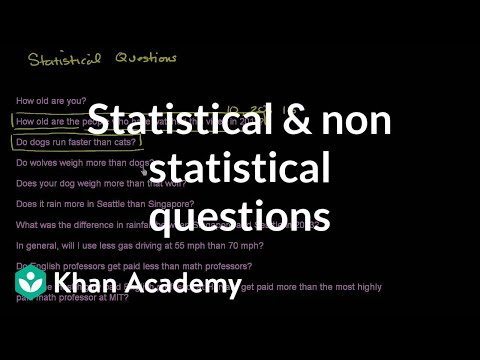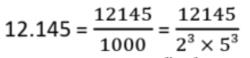Courses

Test: Statistical Tools and Interpretation- Arithmetic Mean, Median and Mode - Assertions & Reason Type Questions

15 Questions MCQ Test Economics Class 11 | Test: Statistical Tools and Interpretation- Arithmetic Mean, Median and Mode - Assertions & Reason Type Questions

Description
Attempt Test: Statistical Tools and Interpretation- Arithmetic Mean, Median and Mode - Assertions & Reason Type Questions | 15 questions in 30 minutes | Mock test for Commerce preparation | Free important questions MCQ to study Economics Class 11 for Commerce Exam | Download free PDF with solutions
QUESTION: 1

Directions : In the following questions, a statement of Assertion (A) is followed by a statement of Reason (R). Mark the correct choice as:Assertion (A): Combined mean is calculated by multiplying the means with their respective frequencies total and then added and divided by the total number of items.Reason (R): In weighted mean, the weights are assigned to the respective items in the series and then the mean is calculated.

Solution: The weighted mean is a type of mean that is calculated by multiplying the weight (or probability) associated with a particular event or outcome with its associated quantitative outcome and then summing all the products together. It is very useful when calculating a theoretically expected outcome where each outcome has a different probability of occurring, which is the key feature that distinguishes the weighted mean from the arithmetic mean.
QUESTION: 2

Directions : In the following questions, a statement of Assertion (A) is followed by a statement of Reason (R). Mark the correct choice as:Assertion (A): For calculating Arithmetic mean, when Cumulative frequencies are given, the frequencies are to be found for the given series or variables.Reason (R): Cumulative frequencies are calculated by adding the frequencies.

Solution: The cumulative frequency will be calculated by adding each frequency from a frequency distribution table to its predecessors. The final value will always be equal to the total for all observations since all frequencies have already been added to the previous total.
QUESTION: 3

Directions : In the following questions, a statement of Assertion (A) is followed by a statement of Reason (R). Mark the correct choice as:Assertion (A): Arithmetic mean is affected by the extreme values.Reason (R): All the values are considered while calculating the mean and so if there are more big values or small values it will affect the calculation of the mean.

Solution: Arithmetic mean has become the most widely used measure of central tendency of data. One demerit of arithmetic means is that it is affected by the extreme value (of observed data). Accordingly, the perfectness of arithmetic mean, as measure of central tendency, is questionable.
QUESTION: 4

Directions : In the following questions, a statement of Assertion (A) is followed by a statement of Reason (R). Mark the correct choice as:

Assertion (A): While calculating Median of the series, the terms need to be arranged in either ascending order or descending order.

Reason (R): In median, the central term is to be found which divides the series into two equal parts.

Solution: Median is a value which divides the series into two equal parts. It is a position which is exactly in the centre, equal number of terms lie on either side of it, when terms are arranged in ascending or descending order.
QUESTION: 5

Directions : In the following questions, a statement of Assertion (A) is followed by a statement of Reason (R). Mark the correct choice as:

Assertion (A): Median is a positional average.

Reason (R): Median is the centre most item in a series arranged in either ascending order or descending order.

Solution: The Median is also known as a positional average. It means that when the data are arranged, median is the middle value if the number of values is odd and the mean of the two middle values, if the number of values is even. Median is a value which divides the series into two equal parts. It is a position which is exactly in the centre, equal number of terms lie on either side of it, when terms are arranged in ascending or descending order.
QUESTION: 6

Directions : In the following questions, a statement of Assertion (A) is followed by a statement of Reason (R). Mark the correct choice as:

Assertion (A): Rahim calculated the value of median to be 16 in the series, 5, 8, 15, 16, 25, 45, 80. He used the formula of odd numbers in this case.

Reason (R): Median for odd series is calculated by the formula ( n + 1 )/2th term.

Solution: To calculate the median first check the number of data set. If the value of ‘n’ is odd use {(n+1)/2}th term and for even ‘n’ value use [(n/2)th term + {(n/2)+1}th term]/2.
QUESTION: 7

Directions : In the following questions, a statement of Assertion (A) is followed by a statement of Reason (R). Mark the correct choice as:

Assertion (A): Mode is dependent on frequencies.

Reason (R): Mode has very little effect on marginal units.

Solution: The value that is most common in a row of data is the mode. In other words, it is the value with the highest frequency. With a distribution in classes is the class with the highest frequency the modal class. If there are two values with the highest frequency, there is no mode.
QUESTION: 8

Directions : In the following questions, a statement of Assertion (A) is followed by a statement of Reason (R). Mark the correct choice as:

Assertion (A): Mode of a series 2, 4, 2, 5, 6, 2, 3, 8, 9, 2, 5, 10 is 2.

Reason (R): Mode of a set of observations is that value which occurs the greatest number of times, i.e., with the highest frequency in a series.

Solution: Any observation occurring maximum number of times or most frequently in a series is the mode of that series. Thus, we can say that the mode is the item having the highest frequency. The most frequently occurring observation in a data set is called Mode (also known as modal value). This is a measure of the tendency of the data values.
QUESTION: 9

Directions : In the following questions, a statement of Assertion (A) is followed by a statement of Reason (R). Mark the correct choice as:

Assertion (A): Mode is affected by the extreme values.

Reason (R): In mode, total cannot be obtained.

Solution: The mode is the value that appears most frequently in a data set. A set of data may have one mode, more than one mode, or no mode at all. Other popular measures of central tendency include the mean, or the average of a set, and the median, the middle value in a set.
QUESTION: 10

Directions : In the following questions, a statement of Assertion (A) is followed by a statement of Reason (R). Mark the correct choice as:

Assertion (A): Mean deviation is calculated in three different ways, namely mean deviation about mean, mean deviation about median and mean deviation about mode.

Reason (R): There are three measures of central tendency that are mean, mode and median.

Solution: Mean is the other name for average. Finding out the mean is very easy, we just have to find the sum of all the numbers and then divide them by the total number of numbers that we have. Mean deviation formula is also a measure of central tendency which can be calculated using Arithmetic Mean, Median, or Mode. It lets us know on average how far all the observations can be from the middle. Each deviation being an absolute value ignores all the negative signs therefore it can rightfully be called an absolute deviation. Also, the deviations must be equal on both sides of the Mean. The mean deviation formula of the observations or values is actually the mean of the absolute deviations from a suitable average. This suitable average can be the mean, median, or mode.
QUESTION: 11

Directions : In the following questions, a statement of Assertion (A) is followed by a statement of Reason (R). Mark the correct choice as:

Assertion (A): A good measure of dispersion needs to be least affected by change in the sampling.

Reason (R): Standard deviation is the best measure of dispersion.

Solution: A measure of dispersion should be rigidly defined. It must be easy to calculate and understand. Not affected much by the fluctuations of observations. Based on all observations.

Standard deviation (SD) is the most commonly used measure of dispersion. It is a measure of spread of data about the mean.

QUESTION: 12

Directions : In the following questions, a statement of Assertion (A) is followed by a statement of Reason (R). Mark the correct choice as:

Assertion (A): Standard Deviation is the best measure of dispersion.

Reason (R): Standard deviation is not based on all the items and is very easy to calculate.

Solution: Standard deviation (SD) is the most commonly used measure of dispersion. It is a measure of spread of data about the mean.
QUESTION: 13

Directions : In the following questions, a statement of Assertion (A) is followed by a statement of Reason (R). Mark the correct choice as:

Assertion (A): Less than or more than ogive can be used to calculate correlation.

Reason (R): When changes in two related variables are in the same ratio, then it is a case of perfect correlation.

Solution:
QUESTION: 14

Directions : In the following questions, a stateme

Assertion (A): Scatter Diagram method is a graphic expression of the degree and direction or absence of correlation.

Reason (R): Scatter Diagram method fails to produce definite trend.

Solution: Scatter diagram offers a graphic expression of the direction and degree of correlation. To construct a scatter diagram, x variables are taken on X-axis and y variable is taken on Y-axis. The cluster of points, plotted, is referred to as a scatter diagram. In this, the degree of closeness of scatter points and their overall direction enables us to examine the relationship.
QUESTION: 15

Directions : In the following questions, a statement of Assertion (A) is followed by a statement of Reason (R). Mark the correct choice as:

Assertion (A): When correlation coefficient (r) is between +0.75 and +1, high degree of positive correlation and when it is between –0.75 to –1 high degree of negative correlation.

Reason (R): The value of correlation lies between + 1 and – 1.

Solution:Use Code STAYHOME200 and get INR 200 additional OFF Use Coupon Code

Track your progress, build streaks, highlight & save important lessons and more!

Similar ContentRelated tests